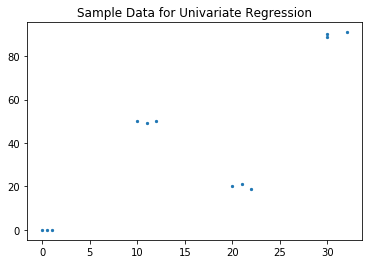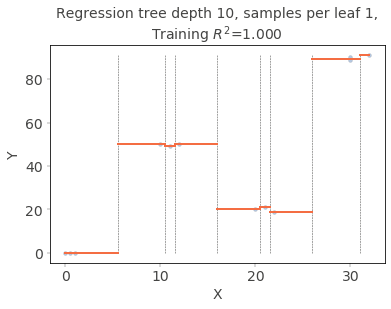# Split Up: dtreeviz (Part 4)

## Goal¶

This post aims to break down the module dtreeviz module step by step to fully understand what is implemented. After fully understanding this, I would like to contribute to this module and submit a pull request.

I really like this module and would like to see this works for other tree-based modules like XGBoost or Lightgbm. I found the exact same issue (issues 15) in github so I hope I could contribute to this issue.

This post is the 4th part: breaking down DTreeViz class and rtreeviz_univar method.

Reference

## DTreeViz class¶

• L23: the beginning of DTreeViz class
• L24-25: __init__ method taking dot object as an input
• L26-78: deal with save, view the visualization as svg file

### rtreeviz_univar¶

• L81: the beginning of rtreeviz_univar method
• L94-102: initial setting for the range of X, y data and converting them into numpy array.
• L104-105: create a scikit-learn decision tree
• L121-122: plot the original X and y data points
• L125-126: plot the vertical line for decision boundary (gray line)
• L128-134: plot the horizontal line for mean line (red line by default)
• L136: Change the appearance of ticks
• L138-140: setting title
• L142-143: setting x and y label based on feature_name and target_name
In :
from pathlib import Path
from graphviz.backend import run, view
import matplotlib.pyplot as plt
from numbers import Number
import matplotlib.patches as patches
import tempfile
import os
from sys import platform as PLATFORM
from colour import Color, rgb2hex
from typing import Mapping, List
from dtreeviz.utils import inline_svg_images, myround
from sklearn import tree
import graphviz

# How many bins should we have based upon number of classes
NUM_BINS = [0, 0, 10, 9, 8, 6, 6, 6, 5, 5, 5]
# 0, 1, 2,  3, 4, 5, 6, 7, 8, 9, 10

def rtreeviz_univar(ax,
x_train: (pd.Series, np.ndarray),  # 1 vector of X data
y_train: (pd.Series, np.ndarray),
max_depth = 10,
feature_name: str = None,
target_name: str = None,
min_samples_leaf = 1,
fontsize: int = 14,
show={'title','splits'},
split_linewidth=.5,
mean_linewidth = 2,
markersize=None,
colors=None):
if isinstance(x_train, pd.Series):
x_train = x_train.values
if isinstance(y_train, pd.Series):
y_train = y_train.values

y_range = (min(y_train), max(y_train))  # same y axis for all
overall_feature_range = (np.min(x_train), np.max(x_train))

t = tree.DecisionTreeRegressor(max_depth=max_depth, min_samples_leaf=min_samples_leaf)
t.fit(x_train.reshape(-1,1), y_train)

splits = []
splits.append(node.split())
splits = sorted(splits)
bins = [overall_feature_range] + splits + [overall_feature_range]

means = []
for i in range(len(bins) - 1):
left = bins[i]
right = bins[i + 1]
inrange = y_train[(x_train >= left) & (x_train <= right)]
means.append(np.mean(inrange))

ax.scatter(x_train, y_train, marker='o', alpha=.4, c=colors['scatter_marker'], s=markersize,
edgecolor=colors['scatter_edge'], lw=.3)

if 'splits' in show:
for split in splits:
ax.plot([split, split], [*y_range], '--', color=colors['split_line'], linewidth=split_linewidth)

prevX = overall_feature_range
for i, m in enumerate(means):
split = overall_feature_range
if i < len(splits):
split = splits[i]
ax.plot([prevX, split], [m, m], '-', color=colors['mean_line'], linewidth=mean_linewidth)
prevX = split

ax.tick_params(axis='both', which='major', width=.3, labelcolor=colors['tick_label'], labelsize=fontsize)

if 'title' in show:
title = f"Regression tree depth {max_depth}, samples per leaf {min_samples_leaf},\nTraining $R^2$={t.score(x_train.reshape(-1,1),y_train):.3f}"
plt.title(title, fontsize=fontsize, color=colors['title'])

plt.xlabel(feature_name, fontsize=fontsize, color=colors['axis_label'])
plt.ylabel(target_name, fontsize=fontsize, color=colors['axis_label'])


### Create a toy sample¶

In :
import numpy as np
import graphviz
from sklearn import tree

X = np.array([0, 1, 0.5, 10, 11, 12, 20, 21, 22, 30, 30, 32]).reshape(-1, 1)
Y = np.array([0., 0, 0, 50, 49, 50, 20, 21, 19, 90, 89, 91]).reshape(-1, 1)
clf = tree.DecisionTreeRegressor(max_depth=3)
clf = clf.fit(X, Y)

plt.scatter(x=X, y=Y, s=5);
plt.title('Sample Data for Univariate Regression');### Visualize a tree using rtreeviz_univar¶

In :
fig, ax = plt.subplots(1)
rtreeviz_univar(ax, pd.Series(X.ravel()), pd.Series(Y.ravel()),
feature_name='X',
target_name='Y',
markersize=15)